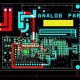# Best Tutorial on Power Electronics?

In this tutorial, we are going to learn about simple tutorial of power electronics.

Power Electronics – Power Electronics deals with how to control and convert power (electric energy) into one form to another and how to utilization of power at high efficiency.

• Below is the distribution of Power Electronics:
• Power – Generation, Transmission, Distribution.
• Electronics – Solid State Device, Electronics Circuit, Signal Process.
• Control – Steady State Characteristics, Dynamic Characteristics.
• Power generation – For Power Generation basically we use below are the process:
• Fossil Fuel
• Nuclear Energy
• Renewable Energy
• Below are the Main Power Electronics Components:
• Thyristor
• Diac
• SCR
• LASCR
• Traic
• UJT
• IGBT
• GTO
• Power Electronics Circuit can be classified into different category which is depending on input and output.
• Rectifier – It is convert AC into DC is called rectifier, It is basically used in battery charger, excitation system of synchronous machines.
• Chopper – It is used for D.C. to D.C. converter which is use in D.C. Drives, Battery Driven vehicles etc.
• AC Voltage Regulators – This is use for AC to AC control and its useful for speed control fans and power pumps.
• Cyclo Converter – It is use for control of slow speed large AC drive.
• Inverter – It is convert DC into AC and it is use in heating induction, UPS, HVDC, wind and solar energy.
• Below are the Power Components which are use in power circuit.
• Power Diode
• Power Transistor
• Power Mosfet
• Power IGBT
• Chopper
• Power Electronic Circuit :
• AC to DC = Rectifier
• DC to DC = Chopper
• AC to AC = AC Chopper
• DC to AC = Inverter
• Uncontrolled – Diode
• Semi Controlled – SCR
• Fully Control – Both ON & OFF are controlled
• Power Circuit Required :
• Power Rating
• Frequency of operating
• Cost and size reduction